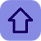# Algorithms and Flowchart in C Programming

Posted by Tushar Bedekar

Algorithms:-
A sequential solution of any program that written in human language,called algorithm.
Algorithm is first step of the solution process, after the analysis of problem, programmer write the algorithm of that problem.
Example of Algorithms:
Q. Write a algorithms to find out number is odd or even?
Ans.
step 1 : start
step 2 : input number
step 3 : rem=number mod 2
step 4 : if rem=0 then
print "number even"
else
print "number odd"
end if
step 5 : stop

Flowchart:-

1. Graphical representation of any program is called flowchart.

2. There are some standard graphics that are used in flowchart as following:

Q. Make a flowchart to input temperature, if temperature is less than 32 then print "below freezing" otherwise print  "above freezing"?
Ans.